# [换票教学]-Asia Miles亚洲万里通 60K Miles玩遍新马泰日台港

「亚洲万里通」Asia Miles奖励换算表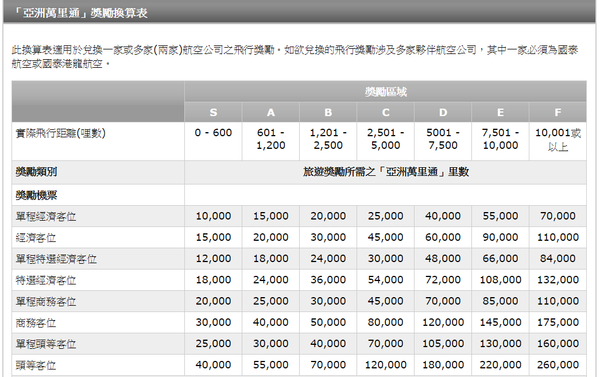「寰宇一家」

「寰宇一家」One Word奖励换算表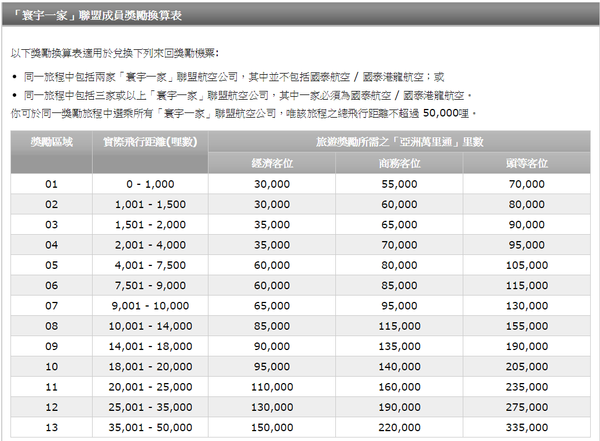###重点来了###

A=>B

A=>B(转机/停留)=>C

A=>B(停留)=>C(停留)=>B(转机)=>A

A=>B(转机)=>C(停留)=>D(停留)=>B(转机)=>A

A=>B(开口) C=>A

A=>B(转机)=>C (目的地开口)D=>E(停留)=>B(转机)=>A

A=>B(转机)=>C(停留)=>D (目的地开口)E=>F(停留)=>B(转机)=>A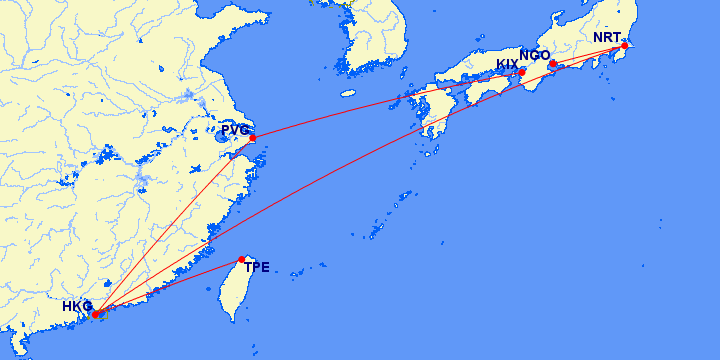TPE-HKG转机-NRT停留-NGO开口,KIX-ICN停留-HKG转机-TPE

(30K经济 50K商务)

HKG要不要转机..都可以看行程安排，因為HKG飞日韩班次较多，比较容易换到票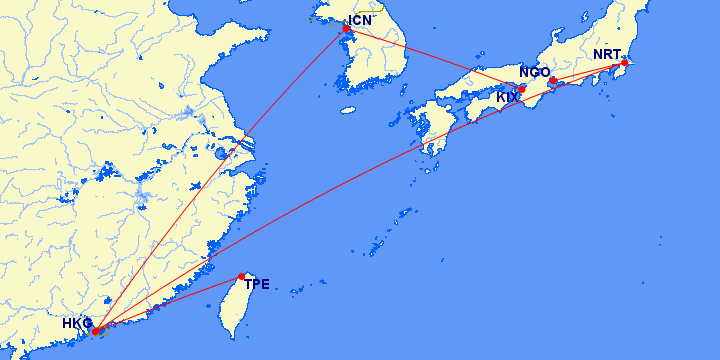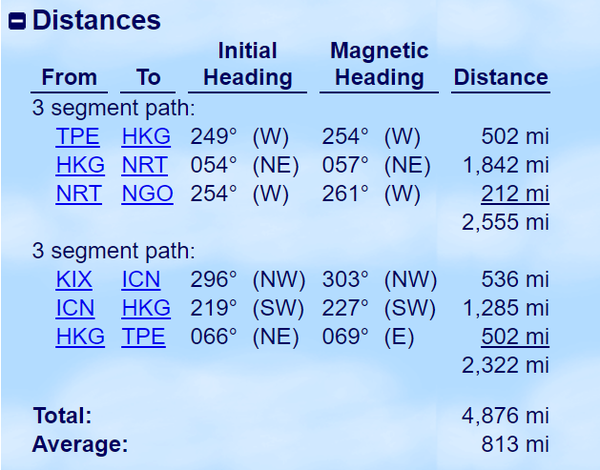A=>B(转机)=>C(停留)=>F(停留)=>B(转机)=>G (出发地开口)

PVG=>NRT(转机)=>CTS(停留)=>KIX(停留)=>HKG(转机)=>XMN(开口)

30K经济 50K商务

SIN=>KUL(停留)=>HKG(转机)=>XMN(开口)PVG=>NRT (开口)KIX=>TPE(停留)=>HKG(转机)=>XMN(停留)=>HKG(停留)=>BKK(停留)=>SIN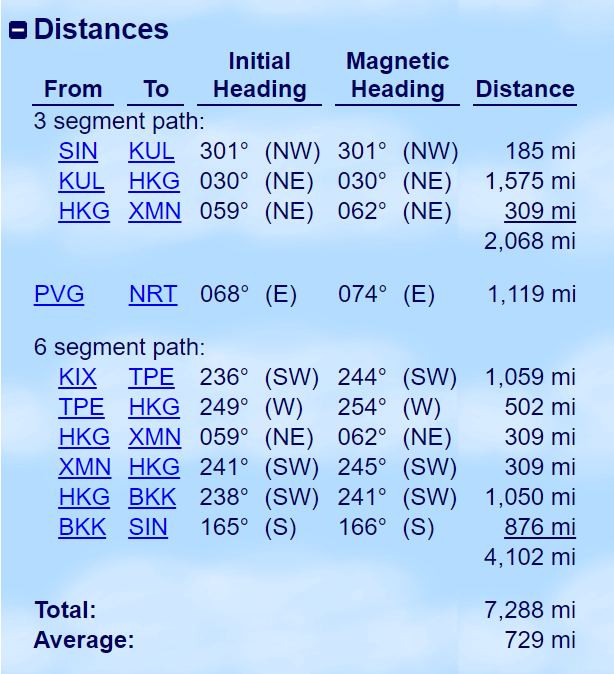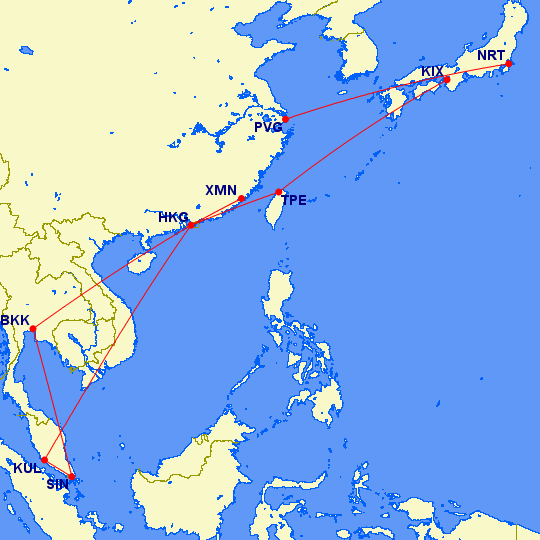「寰宇一家」奖励换算表奖励区域5和6是换经济舱的甜蜜点可以换距离4001-9000mi的行程

1.     首先要先学习如何使用5停留2转机2开口

2.     事先选好停留点并善用转机

3.     善用开口(其实就是一个变相的停留)

4.     安排好航司(两家「寰宇一家」联盟航空公司)或(三家或以上「寰宇一家」联盟航空公司，其中一家必须为CX或KA)

1.可否跳过下一班航班，然后再继续余下旅程？

2.飞行奖励一经签发，可否更改出发日期？

3.飞行奖励一经签发，可否更改行程？

4.机票有效期为多久？可否延长有效期？